Next: Another Useful Lemma Up: Terrestrial Ocean Tides Previous: Useful Lemma

# Transformation of Laplace Tidal Equations

Let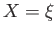,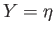,, andin Equations (12.191)-(12.196). It follows that [cf., Equation (12.161)]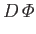(12.202)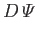(12.203)

where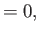(12.204)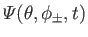(12.205)

Here, use has been made of Equations (12.186) and (12.190). Furthermore [cf., Equations (12.159) and (12.160)],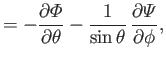(12.206)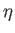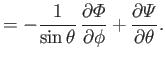(12.207)

Let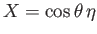,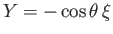,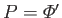, and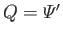in Equations (12.191)-(12.196). It follows that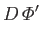(12.208)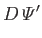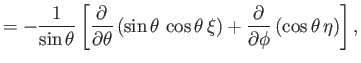(12.209)

where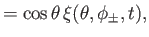(12.210)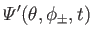(12.211)

Furthermore,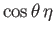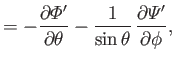(12.212)(12.213)

Substitution of Equations (12.211), (12.212), (12.217), and (12.218) into Equations (12.187) and (12.188) yields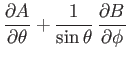(12.214)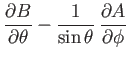(12.215)

where(12.216)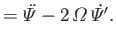(12.217)

Equations (12.219) and (12.220) can be combined to give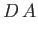(12.218)(12.219)

Moreover, it follows from Equations (12.188), (12.190), (12.209), (12.210), (12.215), and (12.216) that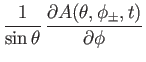(12.220)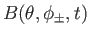(12.221)

We have already seen that the solution of Equation (12.205), subject to the boundary condition (12.204), is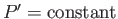. It follows that the solution of Equation (12.223), subject to the boundary condition (12.225), is. Analogous arguments reveal that the solution of Equation (12.224), subject to the boundary condition (12.226), is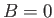. Hence, we deduce that the Laplace tidal equations, (12.186)-(12.188), are equivalent to the following set of equations:(12.222)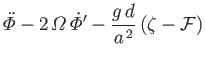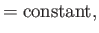(12.223)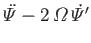(12.224)

where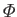,,,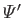, and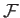are defined in Equations (12.211), (12.212), (12.217), (12.218), and (12.189), respectively.Next: Another Useful Lemma Up: Terrestrial Ocean Tides Previous: Useful Lemma
Richard Fitzpatrick 2016-03-31